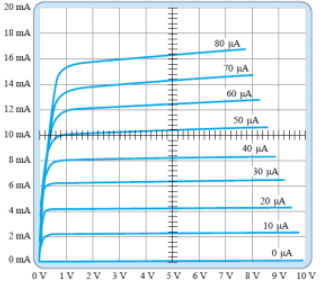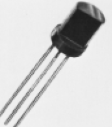Courses

# Test: Limits of Operation

## 10 Questions MCQ Test Analog Circuits | Test: Limits of Operation

Description
This mock test of Test: Limits of Operation for Electronics and Communication Engineering (ECE) helps you for every Electronics and Communication Engineering (ECE) entrance exam. This contains 10 Multiple Choice Questions for Electronics and Communication Engineering (ECE) Test: Limits of Operation (mcq) to study with solutions a complete question bank. The solved questions answers in this Test: Limits of Operation quiz give you a good mix of easy questions and tough questions. Electronics and Communication Engineering (ECE) students definitely take this Test: Limits of Operation exercise for a better result in the exam. You can find other Test: Limits of Operation extra questions, long questions & short questions for Electronics and Communication Engineering (ECE) on EduRev as well by searching above.
QUESTION: 1

### For a BJT, what is typically the shape of the power dissipation curve, if it’s plotted on the output characterisics?

Solution:

Power Dissipation in a BJT is given by P=VCE.IC. This is in the form of k=xy which is the equation of a hyperbola.

QUESTION: 2

### What is the region on the output characteristics below IC = ICEO line called?

Solution:

The region below IC = ICEO is called the cutoff region.

QUESTION: 3

### What is the region on the output characteristics for VCE < VCEsat called?

Solution:

The region below VCE < VCEsat is called the saturation region.

QUESTION: 4

In a BJT amplifier the power gain from base to collector is 4000. The power gain in dB is

Solution:

Gain in dB = 10 log (4000).

QUESTION: 5

Given that the collector power dissipation is 300 mW, what is the value of collector current for the collector to emitter voltage = 12 V?

Solution:

P=VCE.IC => 300mW = (12V)IC => IC=300/12 mA = 25 mA.

QUESTION: 6

Given that the collector power dissipation is 300 mW, what is the value of collector to emitter voltage for collector current = 50 mA?

Solution:

P=VCE.IC => 300mW = VCE(50 mA) => VCE = 300/50 = 6 V.

QUESTION: 7

From the given curve tracer response, what is the value of β for IC = 7 mA and VCE = 5 V?Solution:

From the curve, we get change in IC = (8.2-6.4) mA and change in IB = 10 uA. Hence, /beta; = (1.8/0.01) = 180.

QUESTION: 8

If the positive lead of a DMM, with the mode set to ohmmeter is connected to the base and the negative lead to the emitter and a low resistance reading is obtained, then what is the type of transistor that is being tested?

Solution:

If the positive lead of a DMM, with the mode set to ohmmeter is connected to the base and the negative lead to the emitter and a low resistance reading is obtained, then what is the type of transistor that is being tested is npn.

QUESTION: 9

If the positive lead of a DMM, with the mode set to ohmmeter is connected to the base and the negative lead to the emitter and a high resistance reading is obtained, then what is the type of transistor that is being tested?

Solution:

If the positive lead of a DMM, with the mode set to ohmmeter is connected to the base and the negative lead to the emitter and a low resistance reading is obtained, then what is the type of transistor that is being tested is npn.

QUESTION: 10

For the given transistor, what is the correct sequence of the pins from left to right?Solution:

With the curved side facing away the correct lead sequence is base, collector, emitter.